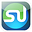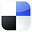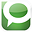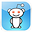## Tuesday, November 9, 2010

### Performing Exponential Smoothing in 3 Steps in Excel

This is one of the following three articles on Time Series Analysis in Excel

Forecasting With Exponential Smoothing in Excel

Forecasting With the Weighted Moving Average in Excel

Forecasting With the Simple Moving Average in Excel

# Performing Exponential Smoothing in 3 Steps in Excel

## Overview of the Moving Average

The moving average is a statistical technique used to smooth out short-term fluctuations in a series of data in order to more easily recognize longer-term trends or cycles. The moving average is sometimes referred to as a rolling average or a running average. A moving average is a series of numbers, each of which represents the average of an interval of specified number of previous periods. The larger the interval, the more smoothing occurs. The smaller the interval, the more that the moving average resembles the actual data series.

Moving averages perform the following three functions:

1. Smoothing the data, which means to improve the fit of the data to a line.

2. Reducing the effect of temporary variation and random noise.

3. Highlighting outliers above or below the trend.

The moving average is one of the most widely used statistical techniques in industry to identify data trends. For example, sales managers commonly view three-month moving averages of sales data. The article will compare a two-month, three-month, and six-month simple moving averages of the same sale data. The moving average is used quite often in technical analysis of financial data such as stock returns and in economics to locate trends in macroeconomic time series such as employment.

There are a number of variations of the moving average. The most-commonly employed are the simple moving average, the weighted moving average, and the exponential moving average. Performing each of these techniques in Excel will be covered in detail in separate articles in this blog. Here is a brief overview of each of these three techniques.

Simple Moving Average

Every point in a simple moving average is the average of a specified number of previous periods. A link to another article in this blog which provides a detailed explanation of the implementation of this technique in Excel is as follows:

http://blog.excelmasterseries.com/2010/10/excels-most-forecasting-tool-simple.html

Weighted Moving Average

Points in the weighted moving average also represent an average of a specified number of previous periods. The weighted moving average applies different weighting to certain previous periods; quite often the more recent periods are given greater weight. A link to another article in this blog which provides a detailed explanation of the implementation of this technique in Excel is as follows:

http://blog.excelmasterseries.com/2010/11/weighted-moving-average-accurate-simple.html

Exponential Smoothing

Points in the exponential moving average also represent an average of a specified number of previous periods. Exponential smoothing applies weighting factors to previous periods that decrease exponentially, never reaching zero. As a result exponential smoothing takes into account all previous periods instead of a designated number of previous periods that the weighted moving average does.

Exponential smoothing is sometimes referred to as an EMA (Exponential Moving Average) or an EWMA (Exponential Weighted Moving Average). There are two versions of a formula for Exponential Smoothing that are commonly used. Exponential smoothing was first suggested by Robert Goodell Brown in 1956. The exponential smoothing that is attributed to him and referred to as “Brown’s Simple Exponential Smoothing” is shown as follows:

S0 = X0

St = αXt + (1-α)St-1

Where

α = Smoothing constant 0 < α < 1

St = Yt-exp = Forecast Value at time = t

Xt = Xt-act = Actual Value at time = t

This formula will be used to perform exponential smoothing in this article. This exponential smoothing formula is convenient because it can be applied when as few as 2 time periods of data are available. It is good practice however to wait until at least several samples are available before performing exponential smoothing. A constant time-series data stream will take approximately 3/α stages to reach 95 percent of the actual value.

The initial value of S0 plays an important role in calculating every subsequent St. Several methods of initializing the value of S0 should be evaluated. One possibility is to set S0 to the target value of the process. Another method is to set S0 to the average of the first four or five actual data values. The smaller the alpha, the greater is the importance of the proper value of S0 because lower value of alpha cause greater smoothing of the forecast and therefore less resemblance to the original data observations.

This simple form of exponential smoothing can also be classified as an Autoregressive Integrated Moving Average (ARIMA) (0, 1, 1) model with no constant term.

Another commonly used version of the exponential smoothing formula is the following:

St = αXt-1 + (1-α)St-1

This formula is attributed to J. Stuart Hunter in 1986. Excel uses this formula for its Exponential Smoothing tool as part of the Data Analysis ToolPak. This article will not cover the use of this formula but will cover the use original exponential smoothing formula, which was the following:

S0 = X0

St = αXt + (1-α)St-1

This formula is referred to as exponential smoothing because it can be shown with algebraic manipulation and direct substitution that the following formula, St = αXt + (1-α)St-1, contains the exponentially decreasing weighted average of all previous data observations.

The following describes the 3-step process of performing exponential smoothing of time-series data in Excel:

## Step 1 – Graph the Original Data in a Time-Series Plot

The line chart is the most commonly-used Excel chart to graph time-series data. An example of such an Excel chart used to plot 13 periods of sales data is shown as follows:

## Step 2 – Create the Exponential Weighted Moving Average With Formulas in Excel

Excel does provide an Exponential Smoothing tool within the Data Analysis menu but it uses a different formula than the original exponential smoothing formula that will be applied in this blog article. The advantage of using a formula instead of a Data Analysis ToolPak tool is that a formula automatically adjusts when data is changed. Further, the formula will automatically recalculate the output if the smoothing factor alpha is changed. A Data Analysis does not automatically recalculate output and must be for each instance that a change is made is made either to data or to alpha. In many cases it is better to use formulas in place of Data Analysis ToolPak tools for those reasons. In cases when a large number of separate formulas working together is required, such as regression or ANOVA, the Data Analysis ToolPak is the preferred solution.

Exponential smoothing can be easily implemented with the following formulas. In this case the smoothing constant alpha is set to 0.2. Alpha can be set to any value between 0 and 1. The closer alpha is to 0, the more smoothing will occur in the forecasted values. The closer alpha is to 1, the less smoothing will occur and consequently the forecasted values will more closely resemble the observed data values.

## Step 3 – Add the Exponential Weighted Moving Average Series to the Chart

This data should now be added to the chart containing the original time line of sales data. The data will simply be added as one more data series in the chart. To do that, right-click anywhere on the chart and a menu will pop up. Hit Select Data to add the new series of data. The moving average series will be added by completing the Edit Series dialogue box as follows:

The chart containing the original data series and that data’s exponential weighted moving average with alpha = 0.2 is shown as follows. Note that the moving average line is quite a bit smoother and raw data’s deviations above and below the trend line are much more apparent. The overall trend is now much more apparent as well.

An exponential weighted moving average with alpha = 0.5 can be created and placed on the chart using nearly the same procedure as follows.

This data should now be added to the chart containing the original time line of sales data along with the 2-interval series. The data will simply be added as one more data series in the chart. To do that, right-click anywhere on the chart and a menu will pop up. Hit Select Data to add the new series of data. The moving average series will be added by completing the Edit Series dialogue box as follows:

As expected less smoothing occurs with the alpha = 0.5 than with alpha = 0.2.

For comparison, an exponential weighted moving average will be calculated with alpha = 0.8 and added to the chart in the same way as follows. Note the progressively increasing alpha caused forecasted values to be closer to actual data observations and less “smoothed.”

This data should now be added to the chart containing the original time line of sales data along with the alpha = 0.2 and alpha = 0.5 series. The data will simply be added as one more data series in the chart. To do that, right-click anywhere on the chart and a menu will pop up. Hit Select Data to add the new series of data. The moving average series will be added by completing the Edit Series dialogue box as follows:

Here is the updated graph withal three exponential weighted moving average data series along with the original data time series.

As expected, the alpha = 0.2 series weighted moving average is significantly smoother than the other two weighted moving averages that have alpha = 0.5 and alpha = 0.8. A smoother graph more closely fits a straight line.

## Analyzing Forecast Accuracy

The two components of forecast accuracy are the following:

Forecast Bias – The tendency of a forecast to be consistently higher or lower than actual values of a time series. Forecast bias is the sum of all error divided by the number of periods as follows:

Bias = ∑Et/n = ∑(Yt-act – Yt-est)/n

A positive bias indicates a tendency to under-forecast. A negative bias indicates a tendency to over-forecast. Bias does not measure accuracy because positive and negative error cancel each other out.

Forecast Error – The difference between actual values of a time series and the predicted values of the forecast. The most common measures of forecast error are the following:

### MAD – Mean Absolute Deviation

MAD calculates the average absolute value of the error and is computed with the following formula:

MAD = ∑ |Et| / n = ∑ |(Yt-act – Yt-est)| / n

Averaging the absolute values of the errors eliminates the canceling effect of positive and negative errors. The smaller the MAD, the better the model is.

### MSE – Mean Squared Error

MSE is a popular measure of error that eliminates the cancelling effect of positive and negative errors by summing the squares of the error with the following formula:

MSE = ∑ Et2 / n = ∑ (Yt-act – Yt-est)2 / n

Large error terms tend to exaggerate MSE because the error terms are all squared. RMSE (Root Square Mean) reduces this problem by taking the square root of MSE.

### MAPE – Mean Absolute Percent Error

MAPE also eliminates the cancelling effect of positive and negative errors by summing the absolute values of the error terms. MAPE calculates the sum of the percent error terms with the following formula:

MAPE = ∑ ( |Et| / Yt-act ) * 100% / n = ∑ ( |(Yt-act – Yt-est)| / Yt-act ) * 100% / n

By summing percent error terms, MAPE can be used to compare forecasting models that use different scales of measurement.

### Calculating Bias, MAD, MSE, RMSE, and MAPE in Excel For the Weighted Moving Average

Bias, MAD, MSE, RMSE, and MAPE will be calculated in Excel to evaluate the 2-interval, 3-interval, and 6-interval weighted moving average forecast obtained in this article and shown as follows:

The first step is to calculate the alpha = 0.2 values of Et, Et2, |Et|, |Et| / Yt-act , and then sum them as follows:

Bias, MAD, MSE, MAPE and RMSE can be calculated as follows:

The same calculations are now performed to calculate Bias, MAD, MSE, MAPE and RMSE for the alpha = 0.5 exponential weighted moving average.

Bias, MAD, MSE, MAPE and RMSE can be calculated as follows:

The same calculations are now performed to calculate Bias, MAD, MSE, MAPE and RMSE for the alpha = 0.8 exponential weighted moving average.

Bias, MAD, MSE, MAPE and RMSE can be calculated as follows:

Bias, MAD, MSE, MAPE and RMSE are summarized for the alpha = 0.2, alpha = 0.5, and alpha = 0.8 exponential weighted moving averages as follows. The alpha = 0.8 exponential weighted moving average is the model that most closely fits that actual data, as would be expected.

*****************************************

Excel Forecasting Tool #3 - Exponential SmoothingIf You Like This, Then Share It...Excel Master Series Blog Directory

Statistical Topics and Articles In Each Topic

• Histograms in Excel
• Bar Chart in Excel
• Combinations & Permutations in Excel
• Normal Distribution in Excel
• t-Distribution in Excel
• Binomial Distribution in Excel
• z-Tests in Excel
• t-Tests in Excel
• Hypothesis Tests of Proportion in Excel
• Chi-Square Independence Tests in Excel
• Chi-Square Goodness-Of-Fit Tests in Excel
• F Tests in Excel
• Correlation in Excel
• Pearson Correlation in Excel
• Spearman Correlation in Excel
• Confidence Intervals in Excel
• Simple Linear Regression in Excel
• Multiple Linear Regression in Excel
• Logistic Regression in Excel
• Single-Factor ANOVA in Excel
• Two-Factor ANOVA With Replication in Excel
• Two-Factor ANOVA Without Replication in Excel
• Normality Testing in Excel
• Nonparametric Testing in Excel
• Post Hoc Testing in Excel
• Creating Interactive Graphs of Statistical Distributions in Excel
• Solving Problems With Other Distributions in Excel
• Optimization With Excel Solver
• Chi-Square Population Variance Test in Excel
• Analyzing Data With Pivot Tables
• SEO Functions in Excel
• Time Series Analysis in Excel

1.2.